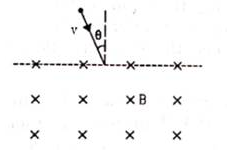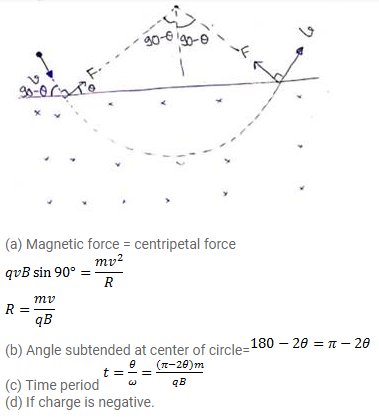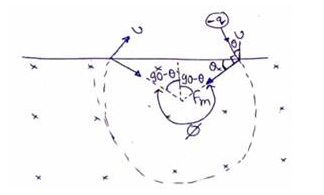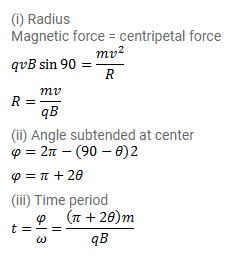# A particle of mass m and positive charge q,

Question:

A particle of mass $m$ and positive charge $q$, moving with a uniform velocity $v$, enters a magnetic field $B$ as shown in figure.

(a) Find the radius of the circular arc it describes in the magnetic field.

(b) Find the angle subtended by the arc at the center.

(c) How long does the particle stay inside the magnetic field?

(d) Solve the three parts of the above problem if the charge q on the particle is negative.Solution: